###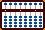Revising Division 278502 ÷ 399 = 698

This is a good revision example because in one instance we revise the first number of the quotient on rod I down to 7 only to find that we must revise it down a second time to 6 in order to compete the first part of the division problem. (See steps 3 - 6 below for clarification.)

Step 1: Set the dividend on rods J through O and the divisor on rods CDE (Fig 1)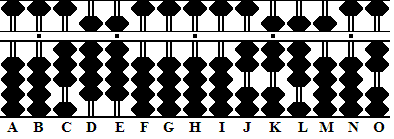Fig. 1 `Step 1` ```A B C D E F G H I J K L M N O . . . . .   0 0 3 9 9 0 0 0 0 2 7 8 5 0 2``` ` `

Step 2:
In looking at the problem it's evident that  27÷ 3 = 9  but in order to continue we need a remainder so we'll go lower and choose 8 as the first number in our answer.

Step 2a: Set 8 on rod I. Multiply 3 x 8 and subtract the product 24 from rods JK (Fig.2)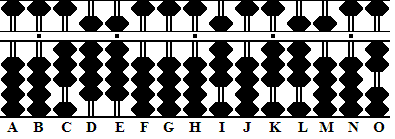Fig. 2 `Step 2` ```A B C D E F G H I J K L M N O . . . . .   0 0 3 9 9 0 0 0 0 2 7 8 5 0 2 (8) Step 2 - 2 4 Step 2a 0 0 3 9 9 0 0 0 8 0 3 8 5 0 2```

We have an error. With only 38 remaining on rods KL we don't have enough to subtract  9 x 8 = 72, in other words we've subtracted 3 from our dividend one too many times. Solution: we must revise both the quotient and the dividend.

Step 3: Reduce the quotient by 1 making it 7.

Step 3a: Continue the revision by adding 3 to the dividend on rod K (Fig 3)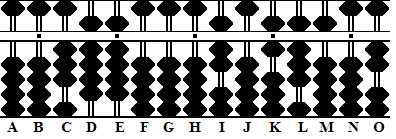Fig. 3 `Step 3` ```A B C D E F G H I J K L M N O . . . . .   0 0 3 9 9 0 0 0 8 0 3 8 5 0 2 - 1 Step 3 + 3 Step 3a 0 0 3 9 9 0 0 0 7 0 6 8 5 0 2```

Step 4:
Having completed the revision in the above step we have 68 on rods KL which this is enough to continue. Multiply 9 x 7 and subtract the product 63 from rods JK (Fig.4)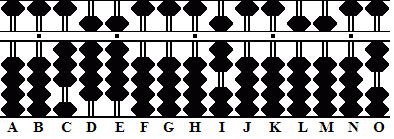Fig. 4 `Step 4` ```A B C D E F G H I J K L M N O . . . . .   0 0 3 9 9 0 0 0 7 0 6 8 5 0 2 (7) - 6 3 Step 4 0 0 3 9 9 0 0 0 7 0 0 5 5 0 2```

Once again we have an error. In order to continue we need to be able to subtract a further 9 x 7 = 63, this time from rods LM. With only 55 available, we've subtracted 39 one too many times from the dividend. Once again,  we must revise both the quotient and the dividend.

Step 5: Reduce the quotient by 1 making it 6.

Step 5a: Continue to revise by adding  39 to the dividend on rods KL (Fig.5)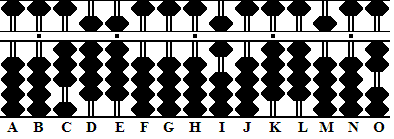Fig. 5 `Step 5` ```A B C D E F G H I J K L M N O . . . . .   0 0 3 9 9 0 0 0 7 0 0 5 5 0 2 - 1 Step 5 + 3 9 Step 5a 0 0 3 9 9 0 0 0 6 0 4 4 5 0 2```

Step 6:
Having completed the revision in the above step we have enough to continue. Multiply 9 x 6 and subtract the product 54 from rods LM (Fig. 6)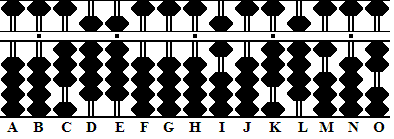Fig. 6 `Step 6` ```A B C D E F G H I J K L M N O . . . . .   0 0 3 9 9 0 0 0 6 0 4 4 5 0 2 (6) - 5 4 Step 6 0 0 3 9 9 0 0 0 6 0 3 9 1 0 2```

Step 7:
For the next number in the quotient it seems reasonable to choose 9. Therefore set 9 on rod J and multiply 9 times each of the numbers in the divisor. Subtract each product from the dividend leaving the remainder on rods LMNO (Fig. 7)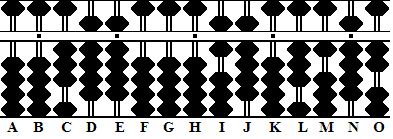Fig. 7 `Step 7` ```A B C D E F G H I J K L M N O . . . . .   0 0 3 9 9 0 0 0 6 9 3 9 1 0 2 (9) - 2 7 Step 7 - 8 1 Step 7a - 8 1 Step 7b 0 0 3 9 9 0 0 0 6 9 0 3 1 9 2```

Step 8:
Once again it looks like 9 might be a good choice for the next answer in the quotient. Set 9 on rod K, multiply 3 x 9 and subtract the product 27 from rods LM (Fig. 8)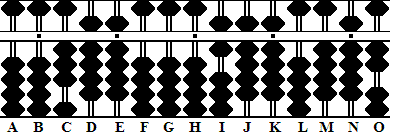Fig. 8 `Step 8` ```A B C D E F G H I J K L M N O . . . . .   0 0 3 9 9 0 0 0 6 9 9 3 1 9 2 (9) - 2 7 Step 8 0 0 3 9 9 0 0 0 6 9 9 0 4 9 2```

Another error has occurred. With only 49 on rods MN we don't have enough to subtract  9 x 9 = 81.

Step 9: Revise by subtracting 1 from the quotient.

Step: 9a: Continue the revision by adding 3 to rod M of the dividend.Fig. 9 `Step 9` ```A B C D E F G H I J K L M N O . . . . .   0 0 3 9 9 0 0 0 6 9 9 0 4 9 2 - 1 Step 9 + 3 Step 9a 0 0 3 9 9 0 0 0 6 9 8 0 7 9 2```

Step 10:
Multiply the revised quotient 9 x 8 and subtract the product 72 from rod MN

Step 10a: Multiply 9 x 8 again and subtract the product 72 from rods NO (Fig.10)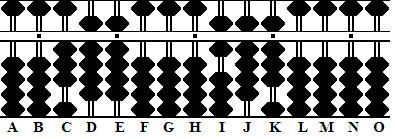Fig. 10 `Step 10` ```A B C D E F G H I J K L M N O . . . . .   0 0 3 9 9 0 0 0 6 9 8 0 7 9 2 (8) - 7 2 Step 10 - 7 2 Step 10a 0 0 3 9 9 0 0 0 6 9 8 0 0 0 0```